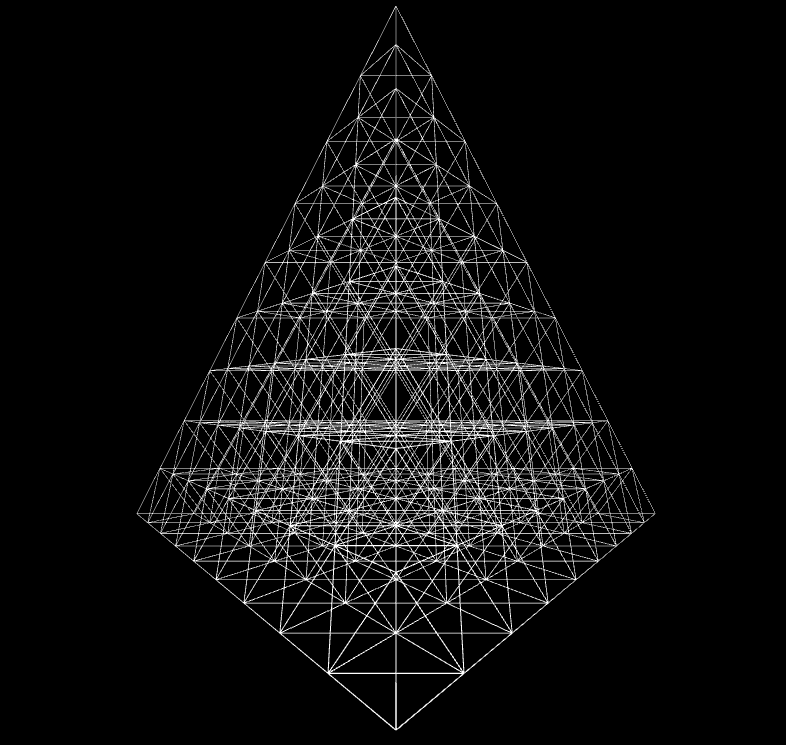## Hannover Fair Pyramids

### Thomas Crevoisier

Based on: Untitled by Georg Nees, 1976

Category: experimental

Description:

An interpretation of this by using a recursive algorithm. This sketch does not run in the browser.```/*
Part of the ReCode Project (http://recodeproject.com)
Based on "Untitled" by Georg Nees
Originally published in "Computer Graphics and Art" v1n2, 1976
*/

/* @pjs pauseOnBlur="true"; */

void setup() {
size(800, 800, P3D);
smooth();
stroke(255);
noFill();
}

void draw() {
background(0);

pushMatrix();
translate(width/2, 10, 0);
branch(10, 60);
popMatrix();
}

void drawPyramid(float xS, float yS, float zS, float h) {

float x1, y1, z1, x2, y2, z2, x3, y3, z3;
//Calculate coordinate of the other points of the pyramid, having the height h of this pyramid and coordinates of the highest point
x1 = xS;
y1 = yS + h;
z1 = zS + h/sqrt(2);

x2 = xS + h*sqrt(6)/4;
y2 = yS + h;
z2 = zS - h*sqrt(2)/4;

x3 = xS - h*sqrt(6)/4;
y3 = yS + h;
z3 = zS - h*sqrt(2)/4;

//Draw the four faces of the pyramids
beginShape();
vertex(xS, yS, zS);
vertex(x1, y1, z1);
vertex(x2, y2, z2);
endShape(CLOSE);
beginShape();
vertex(xS, yS, zS);
vertex(x2, y2, z2);
vertex(x3, y3, z3);
endShape(CLOSE);
beginShape();
vertex(xS, yS, zS);
vertex(x3, y3, z3);
vertex(x1, y1, z1);
endShape(CLOSE);
beginShape();
vertex(x1, y1, z1);
vertex(x2, y2, z2);
vertex(x3, y3, z3);
endShape(CLOSE);
}

void branch(int N, float h) {
N -= 1;

if (N>0) {
//Calculate the next three points which are the other points of the pyramids
float x1, y1, z1, x2, y2, z2, x3, y3, z3;
x1 = 0;
y1 = h;
z1 = h/sqrt(2);

x2 = h*sqrt(6)/4;
y2 = h;
z2 = -h*sqrt(2)/4;

x3 = -h*sqrt(6)/4;
y3 = h;
z3 = -h*sqrt(2)/4;

//Use the recursive function to draw a pyramid for the three points for which we calculate coordinates
pushMatrix();
drawPyramid(0, 0, 0, h);
translate(x1, y1, z1);
branch(N, h);
popMatrix();

pushMatrix();
drawPyramid(0, 0, 0, h);
translate(x2, y2, z2);
branch(N, h);
popMatrix();

pushMatrix();
drawPyramid(0, 0, 0, h);
translate(x3, y3, z3);
branch(N, h);
popMatrix();
}
}```